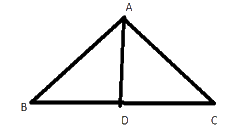# Ex 6.1 Q3 The Triangle and its Properties

## Question

Verify by drawing a diagram if the median and altitude of an isosceles triangle can be same.

Video Solution
Triangle & Its Properties
Ex 6.1 | Question 3

## Text Solution

What is known?

An isosceles triangle.

What is unknown?

If the median and the altitude of an isosceles triangle can be same.

Reasoning:

First, draw an isosceles triangle and draw a line which divides them into two equal parts. The two sides of this triangle are equal because it is an isosceles triangle and the line drawn is perpendicular to the triangle also it divides base into two equal parts.

Steps:

Draw a triangle $$ABC$$ and then draw a line segment $$AD$$ perpendicular to $$BC$$. $$AD$$ is an Altitude of the triangle. It can be observed that length of $$BD$$ and $$DC$$ is also same. Therefore, $$AD$$ is also a median of this Triangle.Learn from the best math teachers and top your exams

• Live one on one classroom and doubt clearing
• Practice worksheets in and after class for conceptual clarity
• Personalized curriculum to keep up with school